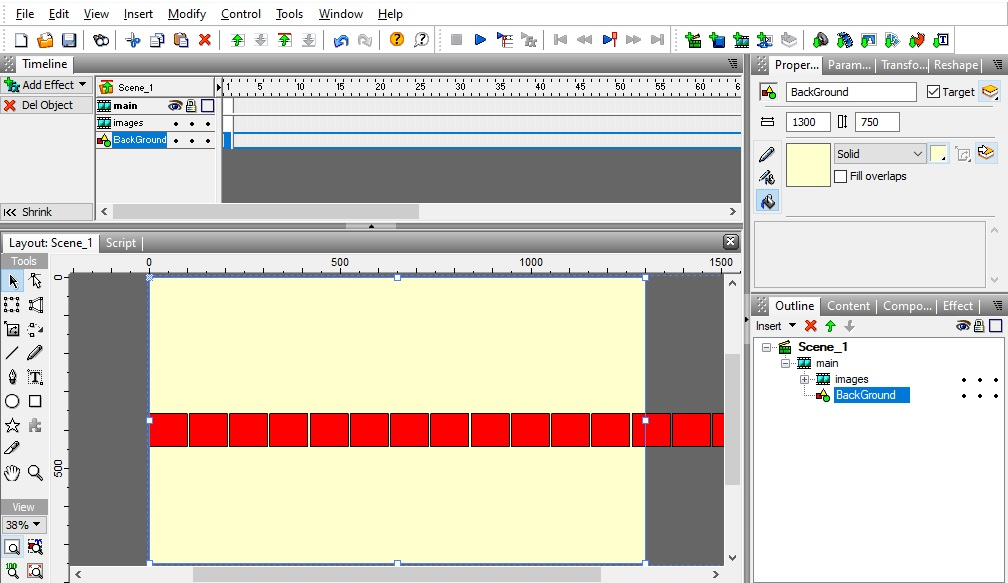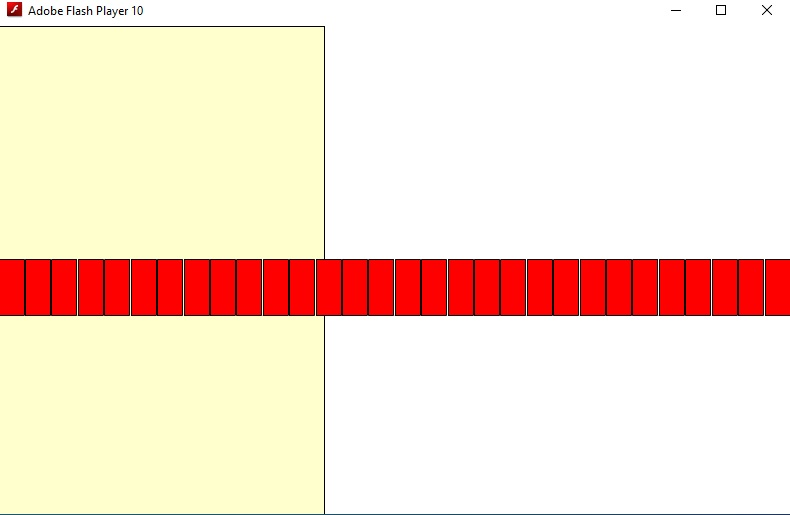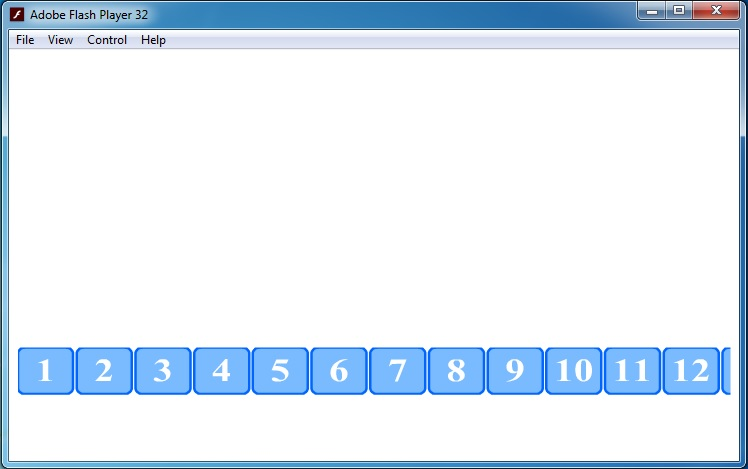A Flash Developer Resource Site

# Thread: A problem in auto scaling

1. ## A problem in auto scaling

hello all

in swishmax

I got very tired of solving auto scaling this fileScene_1 code :

PHP Code:
``` onFrame (2) {         fscommand("ShowMenu",false); stop(); Stage.scaleMode = "noScale"; Stage.align = "TL"; var scaleObject = new Object(); Stage.addListener(scaleObject); scaleObject.onResize = function() { main._x=0; main._width = Stage.width; main._height=Stage.height; if(Stage.width>Stage.height){ main._x=0; main._width=Stage.width; main._height=Stage.height; }else if(Stage.height>Stage.width){ main._x=0;    main._width=Stage.width; main._height=Stage.height; } }; }  ```

but when i test the file , this is the resultfile : https://app.box.com/s/0jpujmfkonr4aqpquqjs859lrcvuo7czReply With Quote

2. Hello,

The accessors for the image contents are:
PHP Code:
``` _root.main.container.mc0//Red square 0. _root.main.container.mc1//Red square 1 etc... _root.main.container.left_btn//Button to slide the images left. _root.main.container.right_btn//Button to slide the images right. _root.main.BackGround//Accessor for the yellow background.  ```
If you want to adjust how many images are showing on the screen as well as the spacing of each square you can modify these values inside of onFrame(2):
PHP Code:
``` var squares_total=10//Amount of squares inside _root.main.container. var squares_visible=4//How many squares to make for the view. var squares_spacing=1.1//Spacing per square.  ```
In the onFrame(2) scripting section:
PHP Code:
``` onFrame (2) {         fscommand("ShowMenu",false); stop(); Stage.align = "TL" //Align the stage to top left. Stage.scaleMode = "noScale"//No scale on the stage movieclips without modification. var scaleObject = new Object(); Stage.addListener(scaleObject); var pane_width=0 var pane_height=0 var squares_total=10//Amount of squares inside _root.main.container. var squares_visible=4//How many squares to make for the view. var squares_spacing=1.1//Spacing per square. var offset=0//Positioning based on _root.main.container.left_btn presses. scaleObject.onResize = function() {//Resize event. resized()//Callable resize function. }; function resized(){    _root.main.BackGround._width=Stage.width//Make the yellow background the same width as the stage.    _root.main.BackGround._height=Stage.height//Make the yellow background the same height as the stage.     if(squares_visible>squares_total){ squares_visible=squares_total } for(var i=0;i<squares_total;i++){//Loop through squares_total inside of _root.main.container. main.container["mc"+i]._width=(Stage.width/squares_visible)/squares_spacing//Modify the width of each image square to be stage width / squares visible / the spacing. main.container["mc"+i]._yscale=main.container["mc"+i]._xscale//Set the y scale of the squares so they match the x scaled width. main.container["mc"+i]._x=(i*main.container["mc"+i]._width*squares_spacing)-(main.container["mc"+i]._width+((offset*main.container["mc"+1]._width*squares_spacing)-main.container["mc"+0]._width)/2)//Reposition each square in the container. } var saved_spacing=((1*main.container["mc"+1]._width*squares_spacing)-main.container["mc"+0]._width)//Amount of spacing per square stored inside a variable. main.container.left_btn._height=main.container["mc"+0]._height//Resize the left slide button. main.container.left_btn._xscale=main.container.left_btn._yscale//Scale the left slide button the the x scaled width. main.container.right_btn._height=main.container["mc"+0]._height main.container.right_btn._xscale=main.container.right_btn._yscale main.container.left_btn._x=-(saved_spacing/2) main.container.right_btn._x=Stage.width-main.container.right_btn._width-saved_spacing/2//Reposition the slide buttons x coordinates main.container._x=saved_spacing/2 //Reposition the x coordinate of the image container. main.container._y=Stage.height/2-main.container._height/2 //Reposition the y coordinate of the image container. } _root.main.container.right_btn.onRelease=function(){//On release mouse event for the right slide button. offset++//Increase offset 1 resized()//Update size & position of the images based on offset. if(main.container["mc"+(squares_total-1)]._x+main.container["mc"+(squares_total-1)]._width<main.container.right_btn._x-main.container.right_btn._width){//If offset is to distant from the last image in the container undo changes. offset--//Decrease offset by 1 } resized()//Update size & position of the images based on offset. } _root.main.container.left_btn.onRelease=function(){//On release mouse event for the left slide button. if(offset>0){//If offset is greater than 0 subtract offset. offset-- } resized()//Update size & position of the images based on offset. } function distanceChecker(a,b){ if(a>b){ return a-b }else{ return b-a } } resized() }  ```Reply With Quote

3. can you make for all time
main.container._y = Stage.height-100 (always )Reply With Quote

4. can you add move to left and move to right by _xmouse ( no butoons needed )Reply With Quote

5. Alright I added the scroll movement based on mouse position, I will change the loop to just container movement to increase performance.

Modify spacing, or visible amount of squares:
PHP Code:
``` var squares_total=10//Squares total. var squares_visible=5//Squares in view. var squares_spacing=1.1//Square spacing.  ```
Modify scroll speed:
PHP Code:
``` var speed:Number=1700  ```
This is the enterFrame function to move left or right:
PHP Code:
``` onEnterFrame=function(){ if(_ymouse<main.container._y+main.container._height&&_ymouse>main.container._y){//If the cursor is inside of the bounds of the container, there can be movement. if(_xmouse>Stage.width/2){//If x mouse is greater than the stage half , scroll the container left. if(main.container["mc"+(squares_total-1)]._x+main.container["mc"+(squares_total-1)]._width-Stage.width>-((1*main.container["mc"+1]._width*squares_spacing)-main.container["mc"+0]._width)){ if(locked_in_right!=offset){ offset+=(((distanceChecker(Stage.width/2,_xmouse)/(Stage.width/2))*100)/speed)//Bi-directional check comparing the cursor location to the stage half / stage half  and than multiplied by 100 to be a full percentage value / by speed variable. resized() } }else{ offset=int(offset) locked_in_right=offset      resized() } } if(_xmouse<Stage.width/2){//If x mouse is less than the stage half , scroll the container right. if(main.container["mc"+0]._x<((1*main.container["mc"+1]._width*squares_spacing)-main.container["mc"+0]._width)&&offset!=0){ offset-=(((distanceChecker(Stage.width/2,_xmouse)/(Stage.width/2))*100)/speed) resized() }else{ offset=0 resized() } } } } resized() }  ```Reply With Quote

6.Reply With Quote

7. I would like the scrolling movement to be continuous
If it reaches the end, it starts again from the same direction
this means
If the movement is to the right and reaches the end, the movement is repeated again in the same direction to the right
If the movement is to the left do the sameReply With Quote

8. i created images scroll

https://app.box.com/s/ny7fndeweo74xh2m5hn0mo586s8shlsk

as you see in this file you can move left and right by xmouseyou can copy this images scroll and past it in ( _root.main ) in your scaling file

i want auto scale this new designed fileReply With Quote

9. Hi,

In the onFrame(2) scripting section, you can add more squares & modify squares_total & the speed settings.
PHP Code:
``` var squares_total:Number=30//main.container.mc0 main.container.mc1 etc... var squares_visible:Number=20//Adjust to display a different amount in view. var squares_spacing:Number=1.1//Adjust to modify spacing. var speed2:Number=50//Adjust to modify speed setting.  ```
In the onFrame(2) scripting section, you can call updateScrollerSettings to resize & reposition the squares based on spacing:
PHP Code:
``` function updateScrollerSettings(){ if(squares_visible<=1){//If the amount in view is less than 0 readjust to have 2 in view. squares_visible=2//Set the value of squares in view to 2. } if(squares_visible>squares_total){//Adjusts the amount of squares in view if the value is exceeding the total amount of squares. squares_visible=squares_total//Set the value of squares in view to squares_total. } for(var i=0;i<squares_total;i++){//Loop through the 30 squares. main.container["mc"+i]._width=(Stage.width/squares_visible)/squares_spacing //Modify the width of the squares in the loop using stage width, spacing setting & amount visible for the view. main.container["mc"+i]._yscale=main.container["mc"+i]._xscale//Modify the yscale to be equal to the x scaled width. main.container["mc"+i]._x=(i*main.container["mc"+i]._width*squares_spacing)-(main.container["mc"+i]._width +  ((offset*main.container["mc"+i]._width*squares_spacing)-main.container["mc"+i]._width))//Modify the x coordinates of each square in the loop using offset for memory when rescaling. main.container2["mc"+i]._width=(Stage.width/squares_visible)/squares_spacing//Do all the same steps for container2. main.container2["mc"+i]._yscale=main.container["mc"+i]._xscale main.container2["mc"+i]._x=(i*main.container2["mc"+i]._width*squares_spacing)-(main.container2["mc"+i]._width +  ((offset*main.container2["mc"+i]._width*squares_spacing)-main.container2["mc"+i]._width)) main.container2["mc"+i]._y=0 } saved_spacing=((1*main.container["mc"+1]._width*squares_spacing)-main.container["mc"+0]._width)//Saved spacing variable to use when separating squares. main.container._x=(saved_spacing/2)//Modify startup view of the first container. main.container._y=Stage.height-main.container._height-saved_spacing/2 maxScroll=main.container._width main.container2._x=main.container._x+main.container._width+saved_spacing//Modify startup coordinates of the second container. main.container2._y=main.container._y }  ```
In the onFrame(2) scripting section, at the function called refreshOffset when you increase variable offset++ you can call this function afterwards to update the position:
PHP Code:
``` function refreshOffset(){//Also works like a resize event. main.BackGround._width=Stage.width//Update background width to stage width. main.BackGround._height=Stage.height//Update background height to stage height. for(var i=0;i<squares_total;i++){//Similar to update scroller settings resize event. main.container["mc"+i]._width=(Stage.width/squares_visible)/squares_spacing main.container["mc"+i]._yscale=main.container["mc"+i]._xscale main.container["mc"+i]._x=(i*main.container["mc"+i]._width*squares_spacing) main.container2["mc"+i]._width=(Stage.width/squares_visible)/squares_spacing main.container2["mc"+i]._yscale=main.container2["mc"+i]._xscale main.container2["mc"+i]._x=(i*main.container2["mc"+i]._width*squares_spacing) } saved_spacing=((1*main.container["mc"+1]._width*squares_spacing)-main.container["mc"+0]._width) main.container._y=Stage.height-main.container._height-saved_spacing/2 main.container2._y=main.container._y if(mode_right=="A"||mode_left=="A"){ main.container._x=(saved_spacing/2) } if(mode_right!=null){//If mode_right is not null it can be "A" to adjust the offset of the scroller to the left. if(distanceChecker(main.container._x,saved_spacing/2)<20&&distanceChecker(main.container._x,saved_spacing/2)>-20&&mode_right=="A"){//First step of mode A position adjustment. main.container._x-=(((main.container["mc"+0]._width/speed2)+saved_spacing/speed2))*offset//Subtract the first container x coordinate. main.container2._x=(main.container._x+main.container._width+saved_spacing)//Modify second container x coordinate to be infront of the first container. } if(distanceChecker(main.container2._x,saved_spacing/2)<20&&distanceChecker(main.container2._x,saved_spacing/2)>-20&&mode_right=="A"){//Second step of mode A position adjustment. main.container2._x=saved_spacing//Placed container 2 to be at a startup view with saved_spacing. main.container._x=main.container2._x+main.container2._width+saved_spacing//Modify the first container to be infront of the second container. mode_right="B"//Set mode to B. offset=0//set offset to 0. } if(mode_right=="B"){//Third step of mode A is now B position adjustment. main.container2._x=saved_spacing/2//Modify container2 to be at x saved_spacing/2 startup view. main.container2._x-=(((main.container["mc"+0]._width/speed2)+saved_spacing/speed2))*offset//Subtract container2 x coordinates to be at the proper multiplied offset. main.container._x=main.container2._x+main.container2._width+saved_spacing//Placed container 1 infront of container 2. } if(main.container._x>saved_spacing&&main.container2._x>main.container._x&&mode_right=="A"){ main.container2._x=(main.container._x-main.container._width-saved_spacing)//Fix any spacing issues. } if(distanceChecker(main.container._x,saved_spacing/2)<20&&distanceChecker(main.container._x,saved_spacing/2)>-20&&mode_right=="B"){//All position adjustment steps completed. mode_right="A"//Set mode back to A. offset=0//Set offset back to A. } } if(mode_left!=null){//Repeat same steps as mode_right. if(distanceChecker(main.container._x,saved_spacing/2)<20&&distanceChecker(main.container._x,saved_spacing/2)>-20&&mode_left=="A"){ main.container._x-=(((main.container["mc"+0]._width/speed2)+saved_spacing/speed2))*offset main.container2._x=(main.container._x-main.container._width-saved_spacing) } if(distanceChecker(main.container2._x,saved_spacing/2)<20&&distanceChecker(main.container2._x,saved_spacing/2)>-20&&mode_left=="A"){ main.container2._x=saved_spacing main.container._x=main.container2._x+main.container2._width+saved_spacing mode_left="B" offset=0 } if(mode_left=="B"){ main.container2._x=saved_spacing/2 main.container2._x-=(((main.container["mc"+0]._width/speed2)+saved_spacing/speed2))*offset main.container._x=main.container2._x+main.container2._width+saved_spacing } if(main.container._x+main.container._width<Stage.width&&main.container2._x<main.container._x&&mode_left=="A"){ main.container2._x=(main.container._x+main.container._width+saved_spacing) } if(distanceChecker(main.container._x,saved_spacing/2)<20&&distanceChecker(main.container._x,saved_spacing/2)>-20&&mode_left=="B"){ mode_left="A" offset=0 } } }  ```
In the onFrame(2) scripting section, at the function called onEnterFrame you said you are having issues with _root._xmouse for your flash version, im using fp10 & fp11 seems to work fine but you might be able to modify it:
PHP Code:
``` onEnterFrame=function(){ if(_root._ymouse<main.container._y+main.container._height&&_root._ymouse>main.container._y){//Check if _ymouse is in bounds of container. if(_root._xmouse>Stage.width/2){//Check if x mouse is greater than the stage half. mode_right="A"//Set mode. mode_left=null//Set left to null. offset++//Increase offset. refreshOffset()//Update offset position. } if(_root._xmouse<Stage.width/2){//Check if x mouse is less than half the stage. mode_right=null//Set mode right to null. mode_left="A"//Set mode. offset--//Decrease offset. refreshOffset()//Update offset position. } } }  ```
Im using your flashplayer version at enterFrame function:
_root._ymouse //Working on fp 10 & fp 11.
_root._xmouse //Working on fp 10 & fp 11.Reply With Quote

10.Reply With Quote

11. Hello, there is a small skipping glitch I fixed:

In the onFrame(2) scripting section:
PHP Code:
``` var error:Number=1;//Room for small decimal error.  ```Reply With Quote

12. PHP Code:
``` onSelfEvent (load) {     mode=true; } onFrame (1) {    import flash.display.*;  this.mc1.mc.removeMovieClip( ) } onFrame (2) {          import flash.display.*;  stop();   function loadBitmapSmoothed(url:String, target:MovieClip) {     var bmc:MovieClip = target.createEmptyMovieClip("bmc",target.getNextHighestDepth());     var listener:Object = new Object();     listener.tmc = target;     listener.onLoadInit = function(mc:MovieClip) {         var mc:MovieClip = mc;         mc._visible = false;         var bitmap:BitmapData = new BitmapData(mc._width, mc._height, true); //------ here how i can to auto fit any image resize---------                 this.tmc._width=77;          this.tmc._height=67;         this.tmc._x=2;         this.tmc._y=2; //-----------------------------------------------------------                 this.tmc.attachBitmap(bitmap, this.tmc.getNextHighestDepth(),"auto", true);         bitmap.bmp.smoothing=true                   mc.forceSmoothing = true         bitmap.draw(mc);          bitmap.bmp.smoothing=true                   mc.forceSmoothing = true     };       var loader:MovieClipLoader = new MovieClipLoader();     loader.addListener(listener);     loader.loadClip(url, bmc); } createEmptyMovieClip("mc1", getNextHighestDepth()); mc1.createEmptyMovieClip("mc", mc1.getNextHighestDepth()); loadBitmapSmoothed("myfolderImages/1.jpg", mc1.mc); mc1.mc.smoothing = true; }  ```

i used this code in ( main.container2.mc0 ) to load external image (100 x 85 ) pixel

1- can you make for loop to do this in all ( mc0 to mc29 )

2- can you edit this code to fit any image resize to ( 100 x 85 ) pixelReply With Quote

13. can you remove ( main.container )Reply With Quote

14. please can you auto scale the file in replay #8Reply With Quote

15. Hello, The movie uses 2 containers to cover both sides.

At the onFrame(2) scripting section, this is the image loader, you can uncomment picture_name++ if you have more pictures in the library:
PHP Code:
``` import flash.display.BitmapData; import flash.net.FileReference; var amount:Number=0 var picture_name:Number=0; import flash.net.FileReference; var loader_listener:Object = new Object(); var img_loader:MovieClipLoader = new MovieClipLoader(); var file_path:String="C:\\Users\\Desktop\\Desktop\\movie\\myfolderImages\\"//Set file path of picture folder. img_loader.addListener(loader_listener); loader_listener.onLoadInit = function(e:MovieClip) {//Load image into both containers. var bitmap:BitmapData = new BitmapData(e._width,e._height, true); main.container["mc"+amount].createEmptyMovieClip("mc3",target.getNextHighestDepth());//Create empty movieclip for container 1. main.container2["mc"+amount].createEmptyMovieClip("mc3",target.getNextHighestDepth());//Create empty movieclip for container 2. main.container["mc"+amount].mc3.attachBitmap(bitmap, main.container["mc"+amount].mc3.getNextHighestDepth(),"auto", true); main.container["mc"+amount].mc3._width=100//Set width & height for the bitmap. main.container["mc"+amount].mc3._height=85 main.container2["mc"+amount].mc3.attachBitmap(bitmap, main.container2["mc"+amount].mc3.getNextHighestDepth(),"auto", true); main.container2["mc"+amount].mc3._width=100 main.container2["mc"+amount].mc3._height=85 bitmap.draw(mc);//Draw bitmap reference. mc._x=0-mc._width//Hide bitmap reference. amount++//Increase amount until it reaches squares_total, equaling 30 image loads. if(amount<squares_total){ _root.img_loader.loadClip(file_path+String(picture_name)+".png",mc);//Load next picture. //picture_name++ //uncomment if more picture names for 0-29, current picture name is 0.png. } } updateScrollerSettings()//Adjust scroller size. createEmptyMovieClip("mc",Stage.getNextHighestDepth());//Create reference movieclip to get drawings from when loading images. _root.img_loader.loadClip(file_path+String(picture_name)+".png",mc);//Load the first image into the container, you can comment this line out to load nothing.  ```
To increase speed you can modify speed2 at the onFrame(2) scripting section:
PHP Code:
``` var saved_spacing:Number=0 var squares_total:Number=30//Adjust amount of squares total. var squares_visible:Number=20//Adjust amount of squares visible. var squares_spacing:Number=1.2//Adjust spacing. var speed2:Number=20//Decrease to increase scroll speed. var error:Number=1;  ```
I modified the for loop to increase performance for the new loadable bitmaps, let me know if more performance is needed, you can also uncomment the last line in the program to load nothing.Reply With Quote

16. You are always great
thank you very much

can you delete load external images function in (Scene_1)

and create this function in ( _root.main.container2)Reply With Quote

17. Ok, if you feel that the swish version is off center by a little at the startup it is because you are using the blue borderline.

I can send you a small donation because it is a better experience to do swish training like that, if you send me your email though.Reply With Quote

18. The swish version will load the bitmaps from inside container2.Reply With Quote

19. thank you very muchReply With Quote

20. I am using bitmaps for this version, it may help with speed.Reply With Quote

####Posting Permissions

• You may not post new threads
• You may not post replies
• You may not post attachments
• You may not edit your posts
•

 » Home » Movies » Tutorials » Submissions » Board » Links » Reviews » Feedback » Gallery » Fonts » The Lounge » Sound Loops » Sound FX » About FK » Sitemap